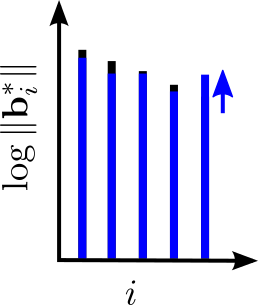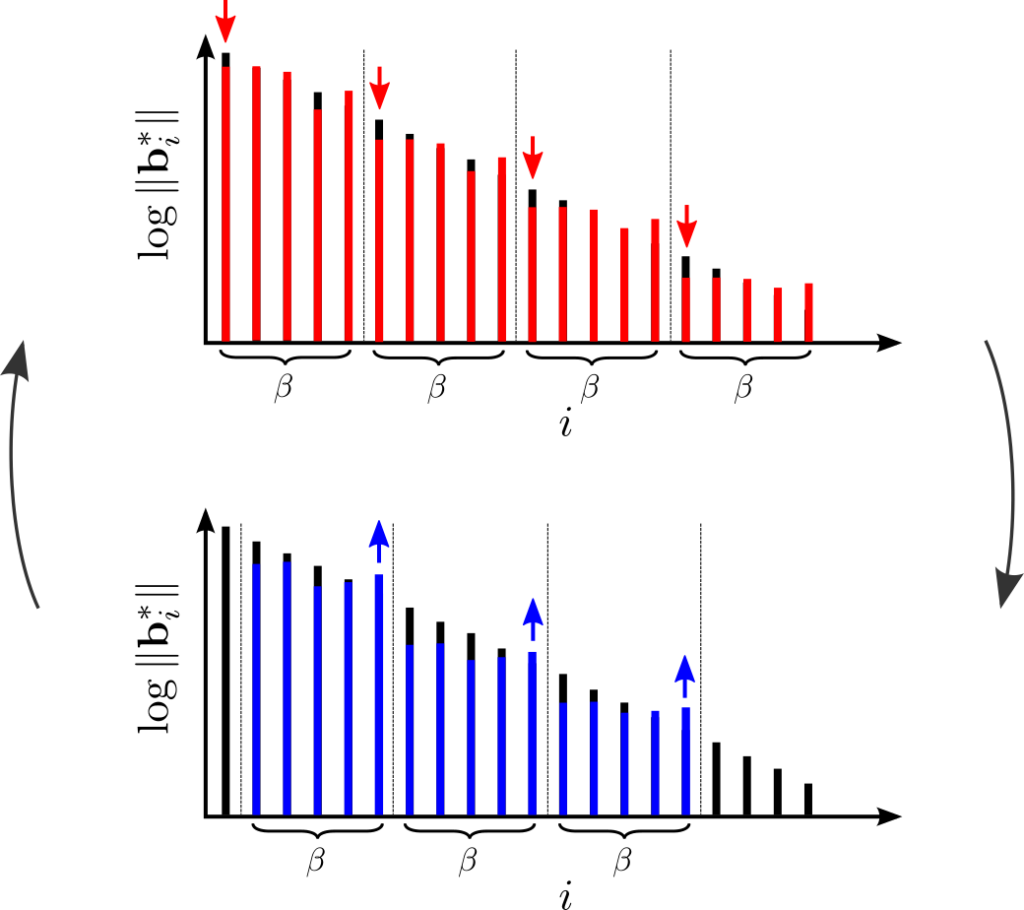# Lattice Blog Reduction – Part II: Slide Reduction

This is the second entry in a series of posts about lattice block reduction. See here for the first part. In this post I will assume you have read the first one, so if you haven’t, continue at your own risk. (I suggest reading at least the first part for context, notations and disclaimers.)

Last time we focused on BKZ which applies SVP reduction to successive projected subblocks. In this post we consider slide reduction, which allows for a much cleaner and nicer analysis. But before we can do that, we need a little more background.

#### A New Tool: Dual SVP Reduction

As you hopefully know, duality is a very useful concept in lattice theory (and in mathematics more generally). It allows to pair up lattices, which are related in a well defined way. Similarly, we can pair up the different bases of two dual lattices to obtain dual bases. I’ll skip the definition of these two concepts since we will not need them. It is sufficient to know that we can compute the dual basis from the primal basis efficiently. One very cool feature of dual bases is that the last vector in the dual basis has a length that is inverse to the length of the last GSO vector of the primal basis. In math: if $${\mathbf{B}}$$ and $${\mathbf{D}}$$ are dual bases, then $$\| {\mathbf{b}}_n^* \| = \| {\mathbf{d}}_n \|^{-1}$$. (If you want to see why this is true at least for full rank lattices, use the fact that in this case $${\mathbf{D}} = {\mathbf{B}}^{-T}$$, and the QR-factorization.) It follows that if $${\mathbf{d}}_n$$ happens to be the shortest vector in the dual lattice, then $${\mathbf{b}}_n^*$$ is as long as possible, since the existence of any basis $$\bar{{\mathbf{B}}}$$ where $$\|\bar{{\mathbf{b}}}_n^*\| > \|{\mathbf{b}}_n^*\|$$ would imply that there exists a dual basis $$\bar {{\mathbf{D}}}$$ such that $$\| \bar{{\mathbf{d}}}_n\| < \| {\mathbf{d}}_n\|$$. By analogy to SVP reduction, we call a basis, where $$\| {\mathbf{b}}^*_n \|$$ is maximized, dual SVP reduced (DSVP reduced). This gives us a new tool to control the size of the GSO vectors: we can apply an SVP algorithm to the dual basis of a projected subblock $${\mathbf{B}}_{[i,j]}$$. This will yield a shortest vector in the dual of this projected sublattice. Then we can compute a dual basis, which contains this shortest vector in the last position and finally compute a new primal basis for this projected subblock, which now locally maximizes $$\|{\mathbf{b}}_j^* \|$$. As we did for primal SVP reduction in the last post, we will assume access to an algorithm that, given a basis $${\mathbf{B}}$$ and indices $$i,j$$, will return a basis such that $${\mathbf{B}}_{[i,j]}$$ is DSVP reduced and the rest of the basis is unchanged. We will call such an algorithm a DSVP oracle. It may sound like this should be somewhat less efficient than SVP reduction, since we have to switch between the dual and the primal bases (which, when done explicitly, requires matrix inversion), but this is not actually the case. In fact, one can implement a DSVP reduction entirely without having to explicitly compute (any part of) the dual basis as shown in [GN08,MW16].Effect of a call to the DSVP oracle. GSO log norms of the input in black, of the output in blue. Note that the sum of the GSO log norms is a constant, so increasing the length of the last vector, decreases the (average of the) remaining vectors.

I hope this figure provides some intuition that such an oracle can be useful. Now let us quantify how much this DSVP oracle helps us. Recall that in the primal SVP reduction we used Minkowski’s theorem to bound the length of the first vector. Since we are now applying the SVP algorithm to the dual, it should come as no surprise that we will use Minkowski’s theorem on the dual lattice, which tells us that $\lambda_1(\widehat{\Lambda}) \leq \sqrt{\gamma_n} \det(\widehat{\Lambda})^{1/n} = \sqrt{\gamma_n} \det(\Lambda)^{-1/n}$ where $$\widehat{\Lambda}$$ is the dual lattice, i.e. the lattice generated by the dual basis. Furthermore, by exploiting above fact that for basis $${\mathbf{B}}$$ and its dual $${\mathbf{D}}$$ we have $$\| {\mathbf{b}}_n^* \| = \|{\mathbf{d}}_n \|^{-1}$$, this shows that if $${\mathbf{B}}$$ is DSVP reduced, i.e. $${\mathbf{d}}_n$$ is a shortest vector in the dual lattice, then $\| {\mathbf{b}}_n^* \| = \|{\mathbf{d}}_n \|^{-1} = \lambda_1(\widehat{\Lambda})^{-1} \geq \frac{\det(\Lambda)^{1/n}}{\sqrt{\gamma_n}}.$ So after we’ve applied the DSVP oracle to a projected block $${\mathbf{B}}_{[i-k+1,i]}$$, we have $\|{\mathbf{b}}^*_i \| \geq \frac{\left(\prod_{j = i-k+1}^{i} \|{\mathbf{b}}_j^* \| \right)^{1/k}}{\sqrt{\gamma_{k}}}.$

# Slide Reduction

Now we have all the tools we need to describe slide reduction [GN08]. One of the major hurdles to apply an LLL-style running time analysis to BKZ seems to be that the projected subblocks considered in that algorithm are maximally overlapping. So slide reduction takes a different route: it applies primal and dual SVP reduction to minimally overlapping subblocks, which allows to still prove nice bounds on the output quality (in fact, even better than BKZ), but also on the running time via a generalization of the LLL analysis. More specifically, let $${\mathbf{B}}$$ be the given lattice basis of an $$n$$-dimensional lattice and $$k$$ be the blocksize. We require that $$k$$ divides $$n$$. (We’ll come back to that restriction later.) Instead of applying our given SVP oracle to successive projected subblocks, we apply it to disjoint projected subblocks, i.e. to the blocks $${\mathbf{B}}_{[1,k]}$$, $${\mathbf{B}}_{[k+1,2k]}$$, etc. So we locally minimize the GSO vectors $${\mathbf{b}}^*_{ik + 1}$$ for $$i \in \{0,\cdots,n/k – 1\}$$. (Technically, we iterate this step with a subsequent LLL reduction until there is no more change, which is important for the runtime analysis, but let’s ignore this for now). So now we have a basis where these disjoint projected subblocks are SVP-reduced. In the next step we shift the blocks by 1 and apply our DSVP oracle to them. (Note that the last block now extends beyond the basis, so we ignore this block.) This has the effect of locally maximizing the vectors $${\mathbf{b}}^*_{ik + 1}$$ for $$i \in \{1,\cdots,n/k – 1\}$$. This might seem counter-intuitive at first, but note that the optimization context for $${\mathbf{b}}^*_{ik + 1}$$ changes between the SVP reduction and the DSVP reduction: $${\mathbf{b}}^*_{ik + 1}$$ is first minimized with respect to the block $${\mathbf{B}}_{[ik+1,(i+1)k]}$$ and then maximized with respect to the block $${\mathbf{B}}_{[(i-1)k+1,ik+1]}$$. So one can view this as using the block $${\mathbf{B}}_{[ik+2, (i+1)k]}$$ as a pivot to lower the ratio between the lengths of the GSO vectors $${\mathbf{b}}^*_{ik+1}$$ and $${\mathbf{b}}^*_{(i+1)k+1}$$. This view is reminiscent of the proof of Mordell’s inequality $$\gamma_n^{\frac{1}{n-1}} \leq \gamma_{n-1}^{\frac{1}{n-2}}$$, which explains the title of the paper [GN08]. The idea of slide reduction is to simply iterate these two steps until there is no more change.Slide reduction in one picture: apply the SVP oracle to the disjoint projected blocks in parallel, then shift the blocks by 1 and apply the DSVP oracle. Repeat.

Let’s dive into the analysis.

#### The Good

When the algorithm terminates, we are guaranteed that the following conditions hold simultaneously:

1. The blocks $${\mathbf{B}}_{[ik+1, (i+1)k]}$$ are SVP reduced for all $$i \in \{0,\cdots,n/k – 1\}$$ (the primal conditions), which implies $\|{\mathbf{b}}^*_{ik+1} \|^{k-1} \leq \gamma_k^{k/2} \prod_{j=ik+2}^{(i+1)k} \|{\mathbf{b}}^*_j \|$ (Note that we raised Minowski’s bound to the $$k$$-th power and canceled one the $$\|{\mathbf{b}}^*_{ik+1} \|$$ on both sides.)

2. The blocks $${\mathbf{B}}_{[ik+2, (i+1)k+1]}$$ are DSVP reduced for all $$i \in \{0,\cdots,n/k – 2\}$$ (the dual conditions), which implies $\gamma_{k}^{k/2} \|{\mathbf{b}}^*_{(i+1)k+1} \|^{k-1} \geq \prod_{j = ik+2}^{(i+1)k} \|{\mathbf{b}}_j^* \|$

(Technically, there is a constant slack factor $$>1$$ involved, which can be set arbitrarly close to 1, but is important for running time. We’ll sweep under the rug for simplicity.)

Just by staring at the two inequalities, you will notice that they can easily be combined to yield: $\|{\mathbf{b}}^*_{ik+1} \| \leq \gamma_k^{\frac{k}{k-1}} \|{\mathbf{b}}^*_{(i+1)k+1} \|$ for all $$i \in \{0,\dots,n/k-2\}$$ and in particular $\|{\mathbf{b}}^*_{1} \| \leq \gamma_k^{\frac{k}{k-1} (\frac{n}{k}-1)} \|{\mathbf{b}}^*_{n-k+1} \| = \gamma_k^{\frac{n-k}{k-1}} \|{\mathbf{b}}^*_{n-k+1} \|$ By a similar trick as last time we can assume that $$\lambda_1({\mathbf{B}}) \geq \|{\mathbf{b}}^*_{n-k+1} \|$$, because the last block is SVP-reduced, which shows that slide reduction achieves an approximation factor $\|{\mathbf{b}}^*_{1} \| \leq \gamma_k^{\frac{n-k}{k-1}} \lambda_1({\mathbf{B}}).$ Done! Yes, it is really that simple. With (very) little more work one can similarly show a bound on the Hermite factor $\|{\mathbf{b}}^*_{1} \| \leq \gamma_k^{\frac{n-1}{2(k-1)}} \det({\mathbf{B}})^{\frac1n}.$ Simply reuse the bounds on the ratios of $$\| {\mathbf{b}}^*_1 \|$$ and $$\| {\mathbf{b}}^*_{ik+1} \|$$ in combination with Minkowski’s bound for each block. (You guessed it: Homework!) Note that both of them are better than what we were able to obtain for BKZ in our last blog post. And in contrast to BKZ one can easily bound the number of calls to the SVP oracle by a polynomial in $$n, k$$ and the bit size of the original basis. The analysis is similar to the one of LLL with a modified potential function: we let $$P({\mathbf{B}}) = \prod_{i=0}^{n/k-2} \det({\mathbf{B}}_{[1,ik]})^2$$. If the basis $${\mathbf{B}}$$ consists of integer coefficients only, this potential is also integral. Furthermore, one can show that if an iteration of slide reduction modifies the basis, it will decrease this potential by at least a constant factor (by using the slack factor we brushed over). This shows that while the basis is modified, the potential decreases exponentially, which results in a polynomial number of calls to the (D)SVP oracle.

We just sketched a complete and elegant analysis of the entire algorithm and it checks all the boxes: best known approximation factor, best known Hermite factor, a polynomial number of calls to its (D)SVP oracle. So what could possibly be bad about it? Remember that we required that the blocksize $$k$$ divides the dimension $$n$$. It seems like it should be easy to get rid of this restriction, for example one could artificially increase the dimension of the lattice to assure that the blocksize divides it. Unfortunately, this and similar approaches will degrade the bound on the output quality – there will be a rounding-up operator in the exponent [LW13]. For small $$k$$ this might not be too much of an issue, but as $$k$$ grows, this results in a significant performance hit. Luckily, a recent work [ALNS19] shows that one can avoid this degradation by combining slide reduction with yet another block reduction algorithm: SDBKZ, which will be the topic of the next post.

#### The Ugly

Slide reduction is beautiful and there is little one can find ugly about it in theory. Unfortunately, experimental studies so far concluded that this algorithm is significantly inferior to BKZ, which (at least to me) is puzzling. This is often attributed to the fact that BKZ uses maximally overlapping blocks, which seems to allow it to obtain stronger reduction notions (even though we cannot prove it). So, one could wonder if there is an algorithm that uses maximally overlapping blocks (and is thus hopefully competetive in practice), but allows for a clean analysis. It turns out that the topic of the next post (SDBKZ) is such an algorithm.

• Gama, Nguyen. Finding short lattice vectors within Mordell’s inequality. STOC 2008

• Li, Wei. Slide reduction, successive minima and several applications. Bulletin of the Australian Mathematical Society 2013

• Micciancio, Walter. Practical, predictable lattice basis reduction. EUROCRYPT 2016

• Aggarwal, Li, Nguyen, Stephens-Davidowitz. Slide Reduction, Revisited—Filling the Gaps in SVP Approximation. https://arxiv.org/abs/1908.03724

## 1 thought on “Lattice Blog Reduction – Part II: Slide Reduction”

This site uses Akismet to reduce spam. Learn how your comment data is processed.